Courses

# IC Engines

## 25 Questions MCQ Test GATE Mechanical (ME) 2022 Mock Test Series | IC Engines

Description
This mock test of IC Engines for GATE helps you for every GATE entrance exam. This contains 25 Multiple Choice Questions for GATE IC Engines (mcq) to study with solutions a complete question bank. The solved questions answers in this IC Engines quiz give you a good mix of easy questions and tough questions. GATE students definitely take this IC Engines exercise for a better result in the exam. You can find other IC Engines extra questions, long questions & short questions for GATE on EduRev as well by searching above.
QUESTION: 1

### 3 cylinder four stroke S.I. engine has stroke length of 900mm and diameter of bore as 900mm. What is the value of cubic capacity of engine?

Solution: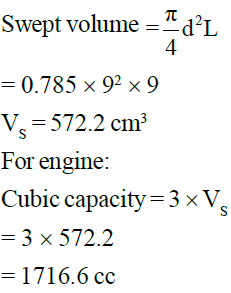QUESTION: 2

### In previous question, clearance volume is given as (compression ratio = 7) : -

Solution: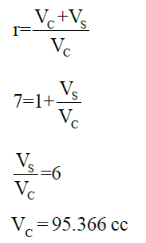QUESTION: 3

### Mechanical efficiency of a certain engine is 75% and it develops a brake power of 40 kW. What will be the new mechanical efficiency at half load if friction power is assumed to be constant?

Solution: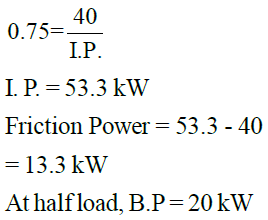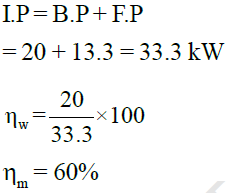QUESTION: 4

Engine has a shaft output of 150 kW and mechanical efficiency 75%. Air consumption is 180 kg/h and air-fuel ratio is 6. If friction loss is reduced by 10 kW, how much amount of fuel will be saved (in kg/ h).
C.V. of fuel = 42 MJ/kg, ηith is assumed to be constant

Solution: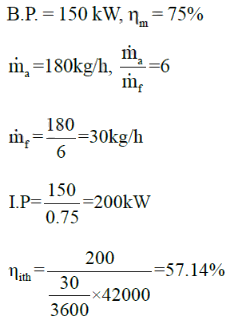Friction loss is reduced by 10 kW

Initial Friction loss = 200 - 150
= 50 kW

New Friction loss = 40 kW
⇒New I.P. = 150 + 40 = 190 kW

B.P is same since load is
unchanged.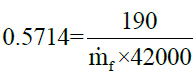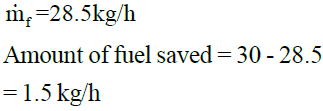QUESTION: 5

Four cylinder, four stroke S.I. engine develops maximum braking torque of 200 N-m at 2500 rpm. At maximum engine torque, brake mean effective pressure is 750 kPa. Diameter of bore is equal to length of stroke. Engine capacity is:-

Solution: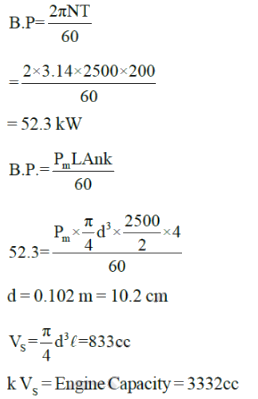QUESTION: 6

Common data for 6 & 7

Single cylinder four stroke C.I. engine running at 2800 rpm has bore diameter of 90mm and stroke length of 120mm. Air consumption is 0.80 kg per minute. Brake power output is 10 kW. Air-fuel ratio is 20:1. Calorific value is 42 MJ/kg. Density of ambient air is 1.18 kg/ m3

Q. Volumetric efficiency is:-

Solution:
QUESTION: 7

Single cylinder four stroke C.I. engine running at 2800 rpm has bore diameter of 90mm and stroke length of 120mm. Air consumption is 0.80 kg per minute. Brake power output is 10 kW. Air-fuel ratio is 20:1. Calorific value is 42 MJ/kg. Density of ambient air is 1.18 kg/ m3.

Q. Brake specific fuel consumption is:-

Solution: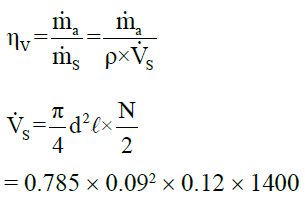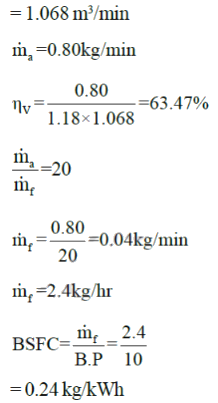QUESTION: 8

Engine has a brake power output of 8kW. Mechanical efficiency of engine is 80%. Calorific value is 40 MJ/kg. ISFC (indicated specific fuel consumption) in kg/kW hr is:-

(Assume indicated thermal efficiency to be 35%)

Solution: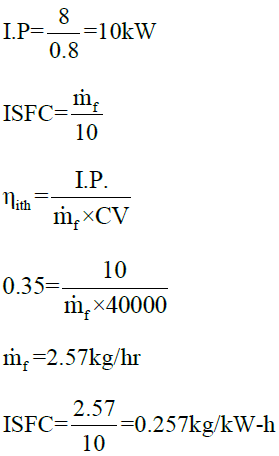QUESTION: 9

Air standard otto cycle has compression ratio of 9. Pressure and temperature at the start of compression process are 101 kPa and 303 K. Isochoric heat addition and heat rejection have values of 1800 kJ/kg and 800 kJ/kg respectively. Mean effective pressure for this cycle is:-

Solution: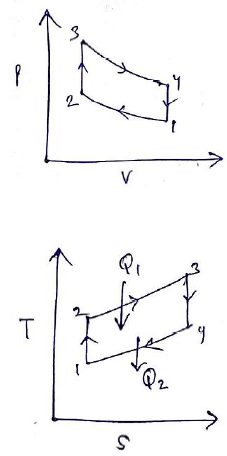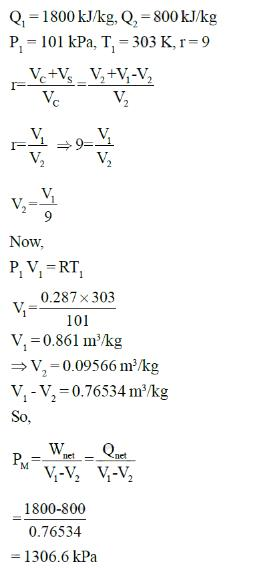QUESTION: 10

An engine works on air standard otto cycle. Engine has bore diameter of 14cm and stroke length of 20 cm.
Isochoric heat addition has a value of 2200 kJ/kg. Clearance volume is 394cc. Value of work output per
cycle per kg of air is:-

Solution: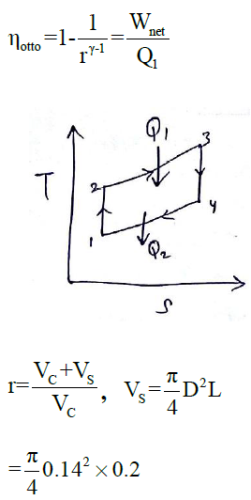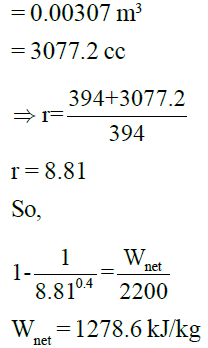*Answer can only contain numeric values
QUESTION: 11

Efficiency of an air standard otto cycle whose clearance volume is 8% of swept volume is ______%.

(Important - Enter only the numerical value in the answer)

Solution: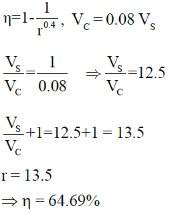QUESTION: 12

Common data for 12 & 13 For stroke S.I engine has four cylinders with clearance volume of 70cm3 each. It has an indicated power of 49 kW and mechanical efficiency of 47%. Engine has stroke length of 10cm and bore diameter of 8cm. Brake thermal efficiency is 45% of air standard efficiency. Calorific value of fuel
is 40 MJ/kg.

(Take N = 2800 rpm).

Engine develops a torque of:-

Solution:
QUESTION: 13

Fuel consumption (in kg/hr) is:-

Solution: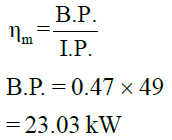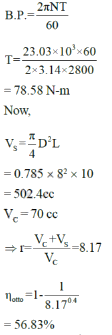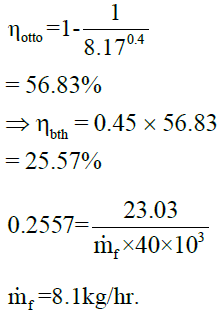QUESTION: 14

Air standard otto cycle operates with volumes of 50cc and 500cc at TDC and BDC respectively.
Thermal efficiency of cycle is:-

Solution:

VC = 50 , VC + VS = 500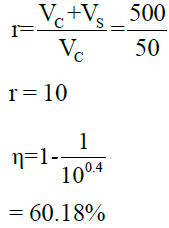QUESTION: 15

In previous question, if power output is 150 kW, then heat input is:-

Solution: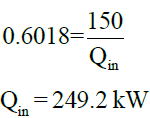QUESTION: 16

If the compression ratio of an engine working on Otto cycle is increased from 5 to 7, the %age increase in efficiency will be

Solution:
QUESTION: 17

Common data for 17 & 18

In a diesel engine, the state of air at the beginning of compression is 280K, 85 kPa. Highest temperature and pressure are 2200K and 6MPa, respectively. Take standard values for air.

Q. Compression ratio is:-

Solution:
QUESTION: 18

Common data for 17 & 18
In a diesel engine, the state of air at the beginning of compression is 280K, 85 kPa. Highest temperature
and pressure are 2200K and 6MPa, respectively. Take standard values for air.

Q. Mean effective pressure is:-

Solution: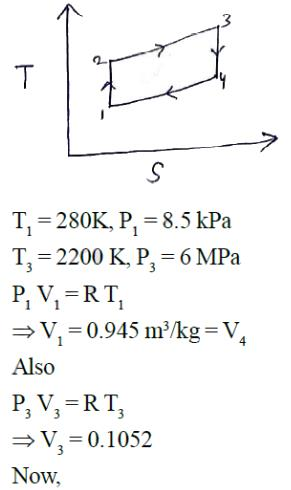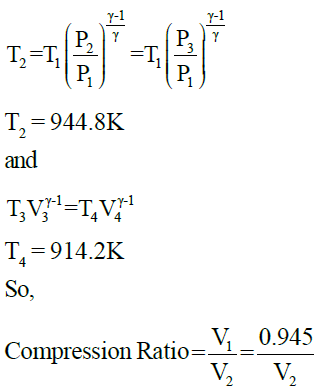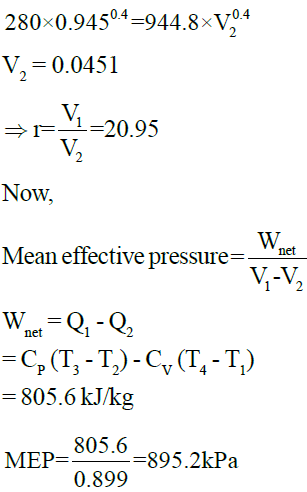QUESTION: 19

Compression ignition engine has compression ratio of 19 when running at 2000 rpm. It has diameter of bore equal to 0.1m and length of stroke 0.11m. Each cycle takes two revolutions and has mean effective pressure of 1400 kPa. It has total of 6 cylinders, the engine horsepower will be:-

Solution: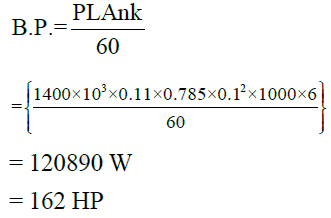QUESTION: 20

Common data for 20 & 21

Compression ratio of a gasoline engine is 9. It receives air at 100C & 100 kPa. Maximum temperature of cycle is 2500K. Use standard properties for air.

Q. Highest cycle pressure is:-

Solution:
QUESTION: 21

Common data for 20 & 21

Compression ratio of a gasoline engine is 9. It receives air at 100C & 100 kPa. Maximum temperature of cycle is 2500K. Use standard properties for air.

Q. Specific energy added during combustion is:-

Solution: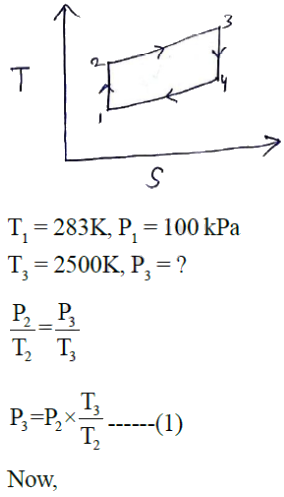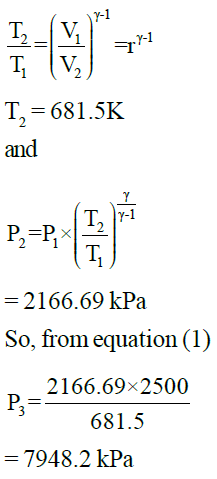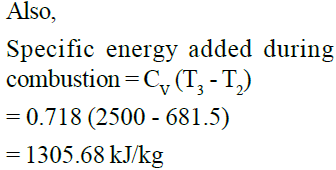QUESTION: 22

Four stroke, four cylinder gasoline engine has compression ratio of 2.3 Litres. Inlet state is 280 K, 70 kPa
and heat addition has value of 1800 kJ/kg during combustion. Engine runs at 2100 rpm, power generated is:-

Solution: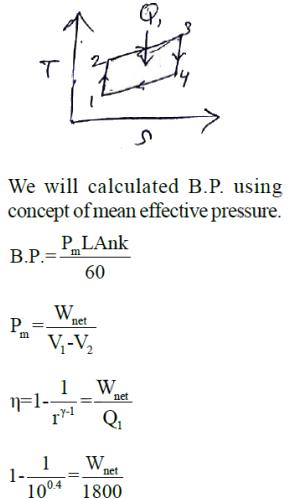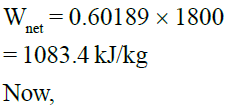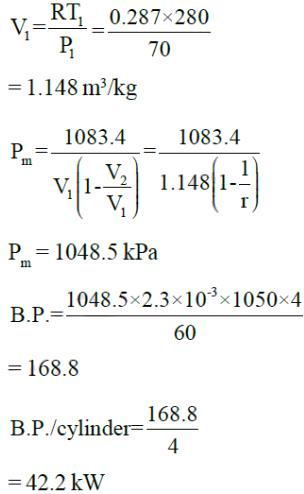QUESTION: 23

Efficiency of diesel cycle will approach to otto cycle when:-

Solution:
QUESTION: 24

Amount of CO2 produced by 1 kg of carbon on complete combustion is:-

Solution: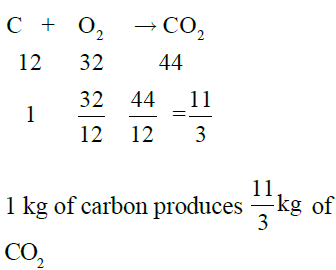QUESTION: 25

Diesel engine knock can be reduced by increasing:-

1. Engine speed

2. Compression ratio

3. Degree of supercharge

4. Injection advance

Which of these are correct?

Solution: